alonsoformula In Spanish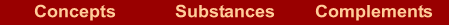What are they?  |  How are they named? If we know the formula  | If we know the name  |  Peroxides  |  Examples  |  Exercises

What are they?

They are compounds formed by a metal and oxygen, in which the oxygen has an oxidation number –2.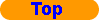How are they named?

For its nomenclature we use the:

Stock´s nomenclature: it is formed by two words. The first word is the name of the metal, followed immediately by the oxidation number between brackets and in Roman numbers. If the oxidation number of the metal is invariable, it is not indicated. The second word is the root of oxygen plus the suffix "–ide"

METAL(N) oxide

The IUPAC also accepts the stoichiometric nomenclature for these oxides, though it is better to use the Stock´s nomenclature when there are metallic atoms and the stoichiometric nomenclature when all the atoms are nonmetals.If we know the formula

In the formula: oxygen has an oxidation number –2, and the oxidation number of the metal can be deduced by knowing that the compound is neutral. If it is always the same, we must know it and it is not necessary to deduce it.

How to determine the oxidation number of the metal?

1. Multiply the oxidation number of oxygen (–2) by its subscript. Change the sign of the result.
2. Divide this result by the subscript of the metal. This it is the value of the oxidation number of the metal, and of the Roman number that you must use.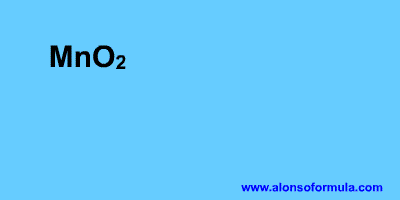If we know the name

In the name: Remember that you must know the symbols of the elements and the oxidation numbers that are invariable.

1. Write the symbol of the first element with the oxidation number; it is either between brackets or you have to know it.
2. Write the symbol of oxygen with an oxidation number –2.
3. Calculate the fewest atoms of each element that you need so that the compound be neutral.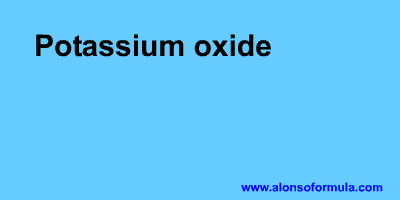Peroxides

There are oxides which have oxygens united by a simple bond (–O–O–), as the oxygenated water or hydrogen peroxide H2O2 (H–O–O–H). The ion dioxide(2–) or ion peroxide, O22–, forms peroxides with elements of the groups 1, 2, 11 and 12.

In these compounds oxygen has an oxidation number –1.

As nearly all the elements of the groups 1, 2, 11 and 12 have an invariable oxidation number , and we must know them, there is no confusion with the oxides of the oxide(2–) ion, O2–. For example:

 Peroxide Stock´s nomenclature Na2O2 Sodium peroxide K2O2 Potassium peroxide MgO2 Magnesium peroxide CaO2 Calcium peroxide Cu2O2 Copper(I) peroxide ZnO2 Zinc peroxideExamples

 Stock´s nomenclature Na2O Sodium oxide MgO Magnesium oxide CaO Calcium oxide Li2O Lithium oxide Ag2O Silver oxide FeO Iron(II) oxide Fe2O3 Iron(III) oxide CrO3 Chromium(VI) oxide TiO2 Titanium(IV) oxide Cu2O Copper(I) oxide CuO Copper(II) oxide ZnO Zinc oxideExercises

In Formulae you have an exercise to write the names of these substances and to check your results. You also have the answer to the exercise.

In Names you have an exercise to write the formulae for these substances and to check your results. You have to introduce the formulae without subscripts, for example for water = H2O. You also have the answer to the exercise.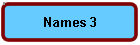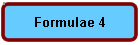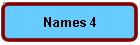Home | Introduction | Oxidation number | Mechanics | Kind of substances | Elementary substances | Metal oxides | Nonmetal oxides | Metal-nonmetal compounds | Nonmetal-nonmetal compounds | Metal hydrides | Hydracids | H with nonmetal | Hydroxides | Oxyacids | Oxysalts | Acid salts | Exercises | Periodic Table | Links
Author: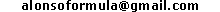WWW.ALONSOFORMULA.COM Formulación InorgánicaFormulación OrgánicaFormulación Inorgánica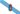Formulación OrgánicaFormulació Inorgánica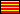Formulació OrgánicaEzorganikoaren Formulazioa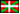Nomenclature of Inorganic Q.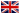Física y Química de ESOFísica e Química de ESOFQ de 1º de BachilleratoFQ de 1º de BacharelatoQuímica de 2º de BachilleratoPrácticas de Química